### Galois invariant basis

Posted on May 29, 2019

Suppose that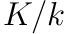is a Galois extension with Galois group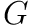. Let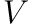be a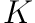vector space with a semi-linear Galois action, i.e., a group homomorphism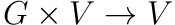denoted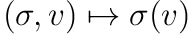such that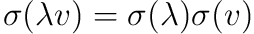for all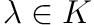and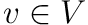. We define the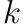-vector space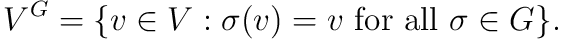Theorem. The natural homomorphism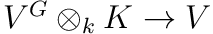given by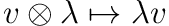is an isomorphism.

To show injectivity it suffices to show that if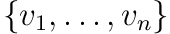are-linearly independent, then they are also-linearly independent. Suppose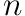is the least integer such that there exists a-linearly independent setand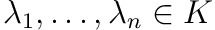such thatBy dividing by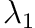we may assume that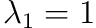. Moreover, sinceis-linearly independent, we must have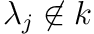for some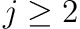. Thus there exists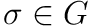such that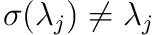. Observe thatTherefore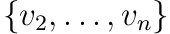is a smaller-linearly independent set that is not-linearly independent, a contradiction to our selection ofas the smallest number of vectors needed for such an occurrence.

It remains to show that the map is surjective. Let us pick a-basis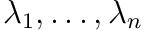forand write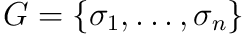with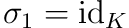. Define the matrixObserve that each column defines a character ofwith values ingiven by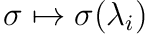. Thus the columns are linearly independent by linear independence of characters. So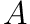is an invertible matrix with inverse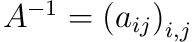. For anylet us also defineNow let us pick a fixed. Observe we have the equation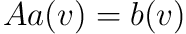given explicitly byMultiplying both sides on the right by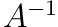gives us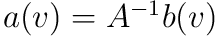. In particular, we haveWe finally observe that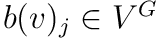for each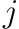as each is invariant under the action of. Thus the map is surjective.

The inspiration for the above proof comes from (1, Proposition 1.2.2). Different proofs of the statement can be found in (2, Lemma II.5.8.1) or (3, Lemma 2.3.8).

1. Nicolas Garrel, An introduction to Galois cohomology through central simple algebras, 2014
2. Joseph Silverman, The arithmetic of elliptic curves, 2nd ed., Springer Graduate Texts in Mathematics, 1992
3. Gille Szamuely, Central simple algebras and galois cohomology, Cambridge Studies in Advanced Mathematics, 2006.